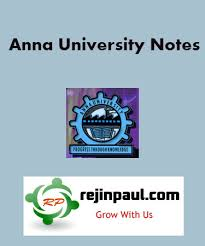### New Update

6/recent/ticker-posts

# EC8251 Circuit Analysis Syllabus Notes Question Bank with Answers Anna University

## EC8251 Circuit Analysis Syllabus Notes Question Paper Question Banks Anna University - Regulation 2017 2nd Semester Notes CBCS

Anna University Circuit Analysis Syllabus Notes Question Bank Question Papers

Anna University EC8251 Circuit Analysis Notes are provided below. EC8251 Notes all 5 units notes are uploaded here. here EC8251 Circuit Analysis notes download link is provided and students can download the EC8251 Lecture Notes and can make use of it.EC8251 Circuit Analysis Syllabus Regulation 2017
UNIT I BASIC CIRCUITS ANALYSIS AND NETWORK TOPOLOGY
Ohm’s Law – Kirchhoff’s laws – Mesh current and node voltage method of analysis for D.C and A.C. circuits - Network terminology - Graph of a network - Incidence and reduced incidence matrices – Trees –Cutsets - Fundamental cutsets - Cutset matrix – Tie sets - Link currents and Tie set schedules -Twig voltages and Cutset schedules, Duality and dual networks.
UNIT II NETWORK THEOREMS FOR DC AND AC CIRCUITS
Network theorems -Superposition theorem, Thevenin’s theorem, Norton’s theorem, Reciprocity theorem, Millman’s theorem, and Maximum power transfer theorem ,application of Network theorems- Network reduction: voltage and current division, source transformation – star delta conversion.
UNIT III RESONANCE AND COUPLED CIRCUITS
Resonance - Series resonance - Parallel resonance - Variation of impedance with frequency -Variation in current through and voltage across L and C with frequency – Bandwidth - Q factor -Selectivity. Self inductance - Mutual inductance - Dot rule - Coefficient of coupling - Analysis of multiwinding coupled circuits - Series, Parallel connection of coupled inductors - Single tuned and double tuned coupled circuits.
UNIT IV TRANSIENT ANALYSIS
Natural response-Forced response - Transient response of RC, RL and RLC circuits to excitation by Step Signal, Impulse Signal and exponential sources - Complete response of RC, RL and RLC Circuits to sinusoidal excitation.
UNIT V TWO PORT NETWORKS
Two port networks, Z parameters, Y parameters, Transmission (ABCD) parameters, Hybrid(H) Parameters, Interconnection of two port networks, Symmetrical properties of T and π networks.